### Home > CALC > Chapter 10 > Lesson 10.4.1 > Problem10-136

10-136.
1. Determine if the following series converge absolutely, converge conditionally, or diverge. Be sure to indicate which convergence test you used. Homework Help ✎

1.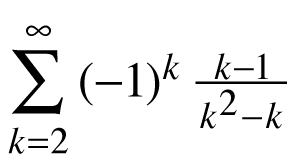2.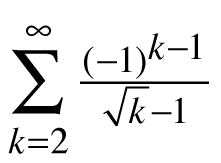3.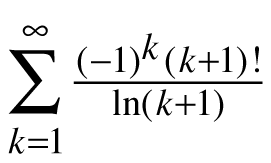4.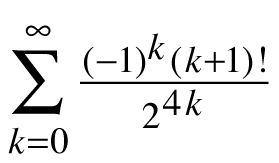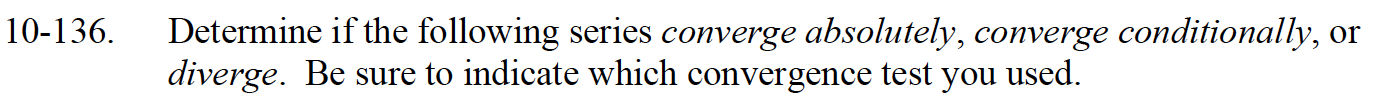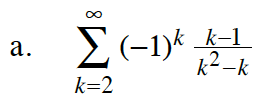$\sum_{k=1}^{\infty}\Big|(-1)^k\frac{k-1}{k^2-k}\Big|=\sum_{k=1}^{\infty}\frac{k-1}{k(k-1)}$

$=\sum_{k=1}^{\infty}\frac{1}{k}\text{ which diverges}$

Therefore series does not converge absolutely.

Does this series converge conditionally?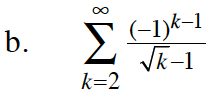$\lim_{k\to\infty}\frac{1}{\sqrt{k}-1}=0$

Therefore this series converges conditionally by the Alternating Series Test.

Does this series converge absolutely?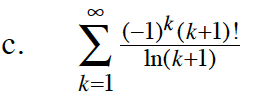$\lim_{k\to\infty}\frac{(k+1)!}{\ln(k+1)}=\infty$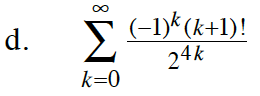$\lim_{k\to\infty}\frac{(k+1)!}{2^{4k}}=?$